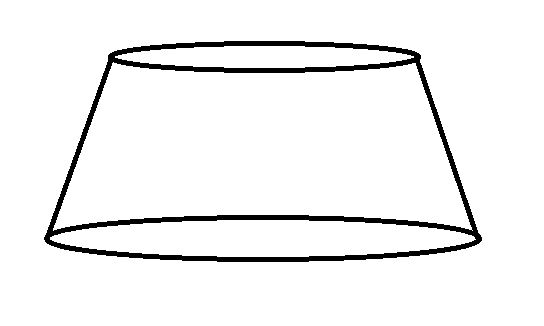# Create truncated Cone

Hello,

I want to display a truncated code like the followingAny hint would be appreciated.
Thanks

You can create any rotationally symmetric shape from a polyline using `vtkRotationalExtrusionFilter`.

This example shows how to use the `vtkRotationalExtrusionFilter` to extrude a line segment, so is directly applicable for generating this shape:

https://vtk.org/gitweb?p=VTK.git;a=blob;f=Filters/Modeling/Testing/Cxx/TestRotationalExtrusion.cxx

1 Like

Also look at: CappedSphere. You should be able to easily modify the function GetLine to do what you want.

1 Like

I made a vtkTruncatedConeSource with a simple modification of vtkCylinderSource. Just add some getters and setters for Radius1 and Radius2 instead of Radius. And replace the usage of Radius in the top and bot values in vtkCylinderSource.cxx with Radius1 and Radius2 appropriately. I’d post the whole code but vtkCylinderSource.h/.cxx is a lot.

That should work, although creating a line and rotating it would have been simpler.Here is a quick example creating a line to rotate to make a truncated cone that you can drop into CappedSphere (the C++ version is easy to create from this, it is left as an exercise for the reader).

``````def get_line1(height, radius1, radius2, step, uncapped):
"""
Get the points for a line.

The parametric equations for the line are:
x = x1 + (x2 - x1) / t
z = z1 + (z2 - z1) / t
0 <= t <= 1

Conditions:
height > 0
step > 0

:param height: Height of the cone.
:param step: The number of points in each line.
:param uncapped: True if uncapped.
:return: A vector of points.
"""
pts = list()

t = 0
z02 = height / 2
if not uncapped:
# Create the cap for radius2
while t < 1:
if t != 0:
x = x02 * t
z = z02
else:
x = 0
z = z02
pt = (x, 0, z)
pts.append(pt)
t += 1.0 / step
# The sloped line for the cone
t = 0
z21 = height / 2
while t <= 1:
if t != 0:
x = radius2 + x21 * t
z = z21 - height * t
else:
z = z21
pt = (x, 0, z)
pts.append(pt)
t += 1.0 / step
t = 0
z10 = -height / 2
if not uncapped:
# Create the cap for radius1
while t < 1:
if t != 0:
x = radius1 - x10 * t
z = z10
else: